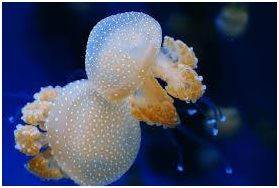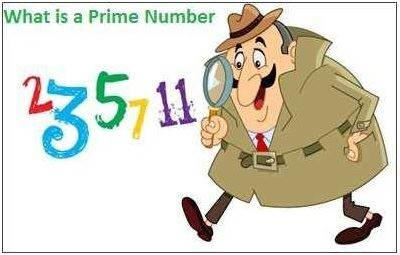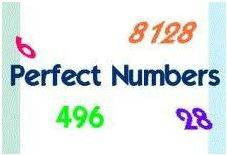# Different Types of Numbers

PRIME, COMPOSITE, PERFECT AND CO-PRIME NUMBERS

Did you know that among marine animals, jellyfish is one such creature which does not have a brain or a heart? Did you know that unlike all other mammals, the blood of a shark, Carcharhinus is colourless?These are some of the interesting exceptions you find in the world around you. In the same way, in the world of numbers too, we have many exceptions. Let us find out what these exceptions are :

PRIME NUMBERS

Those positive numbers that do not have any other divisors, other than 1 and the number itself, are called prime numbers. For example: 7 . Let’s find out what its divisors are: 1 and 7. That is it. Number 7 does not have any other divisors, than 1 and the number itself. Such numbers are called prime numbers.• 1 is neither a prime number nor a composite number.
• 0 is not a prime or composite number, because it is neither a positive or negative number.

Prime numbers between 2 and 100

2, 3, 5, 7, 11, 13, 17, 19, 23,29, 31, 37, 41, 43, 47, 53, 59, 61, 67, 71, 73, 79, 83, 89 and 97

Prime numbers between 100 and 500

• 101, 103, 107, 109, 113, 127, 131, 137, 139, 149, 151, 157, 163, 167, 173, 179, 181, 191, 193, 197, 199
• 211, 223, 227, 229, 233, 239, 241, 251, 257, 263, 269, 271, 277, 281, 283, 293
• 307, 311, 313, 317, 331, 337, 347, 349, 353, 359, 367, 373, 379, 383, 389, 397
• 401, 409, 419, 421, 431, 433, 439, 443, 449, 457, 461, 463, 467, 479, 487, 491, 499

Remembering at least the prime numbers between 2 and 100 can be very helpful. There is no particular formula to derive prime numbers. They have to be learnt otherwise.

COMPOSITE NUMBERS

Now that we have learnt what prime numbers are, we should know what composite numbers are. Composite numbers are the exact opposite of prime numbers.

All those positive digits which have at least one more divisor other than 1 and itself are called composite numbers.

For example 18 is a composite number. This is because 18 has the following divisors: 1, 2,9,3,6, and 18. 14 is also a composite number. This is because 14 has divisors 1 , 2, 7 and 14.

• These are the composite numbers between 2 and 100 :

4, 6, 8, 9, 10, 12, 14, 15, 16, 18, 20, 21, 22, 24, 25, 26, 27, 28, 30, 32, 33, 34, 35, 36, 38, 39, 40, 42, 44, 45, 46, 48, 49, 50, 51, 52, 54, 55, 56, 57, 58, 60, 62, 63, 64, 65, 66, 68, 69, 70, 72, 74, 75, 76, 77, 78, 80, 81, 82, 84, 85, 86, 87, 88, 90, 91, 92, 93, 94, 95, 96, 98, 99, 100, 102, 104, 105, 106, 108

PERFECT NUMBERS

A number that is equal to the sum of its positive divisors except the number itself is called a perfect number. For example: 6 is a perfect number, because; 6=1+2+3

A perfect number can also be defined as one which is equal to half the sum of all its dividers, including the number itself. For example : 6 = (1+2+3+6)/2

The next 3 perfect numbers are 28 = 1+2+4+7+14 ; 496 and 8128CO-PRIME NUMBERS

Two integers are said to be co-prime if they don’t have any common factors.

For example, 7 and 45 are co-prime numbers since they don’t have any factors in common. But 8 and 62 are not co-prime numbers since they have the common factor 2.

• Prime numbers are always co-prime to each other.
• Any two consecutive numbers are co-prime. For example, 14, 15. These two numbers don’t have any common factor among them and are co-prime numbers.

Now that you know what different types of numbers are, you will have a better grip on different areas in Maths.

Fall in love with learning at BYJU’S